Скачать презентацию MARKET DEMAND CONSUMER SURPLUS OWN-PRICE ELASTICITY

2fa78837d731b68b7e64cf034044598c.ppt

• Количество слайдов: 35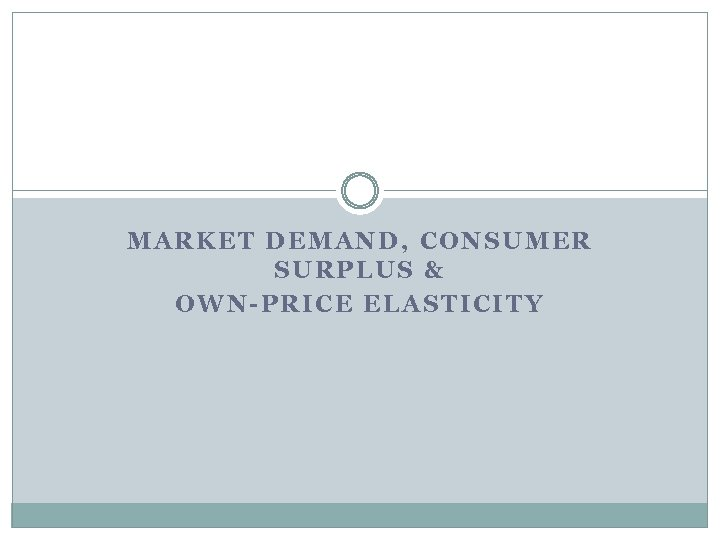MARKET DEMAND, CONSUMER SURPLUS & OWN-PRICE ELASTICITY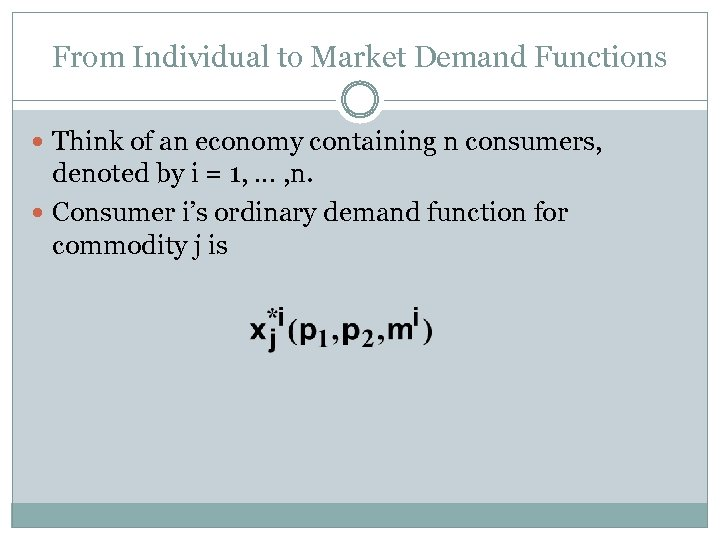From Individual to Market Demand Functions Think of an economy containing n consumers, denoted by i = 1, … , n. Consumer i’s ordinary demand function for commodity j is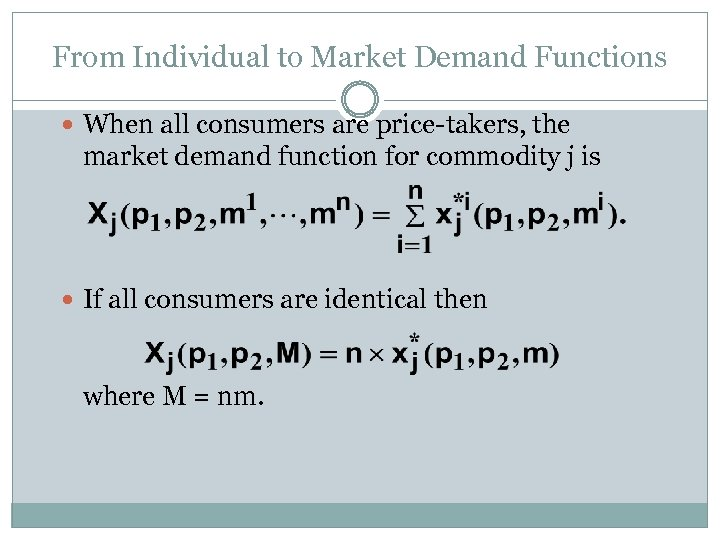From Individual to Market Demand Functions When all consumers are price-takers, the market demand function for commodity j is If all consumers are identical then where M = nm.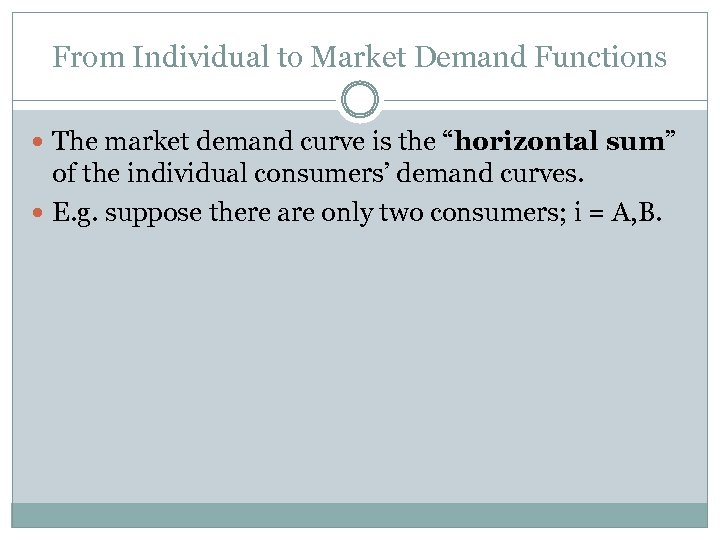From Individual to Market Demand Functions The market demand curve is the “horizontal sum” of the individual consumers’ demand curves. E. g. suppose there are only two consumers; i = A, B.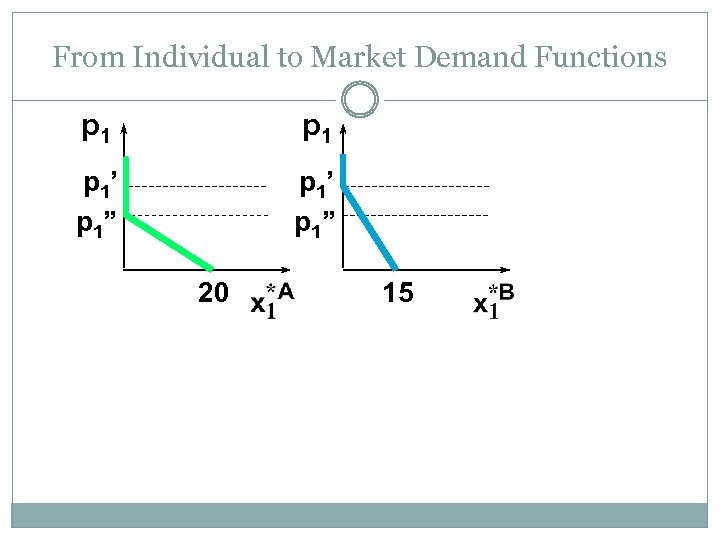From Individual to Market Demand Functions p 1 p 1’ p 1” 20 15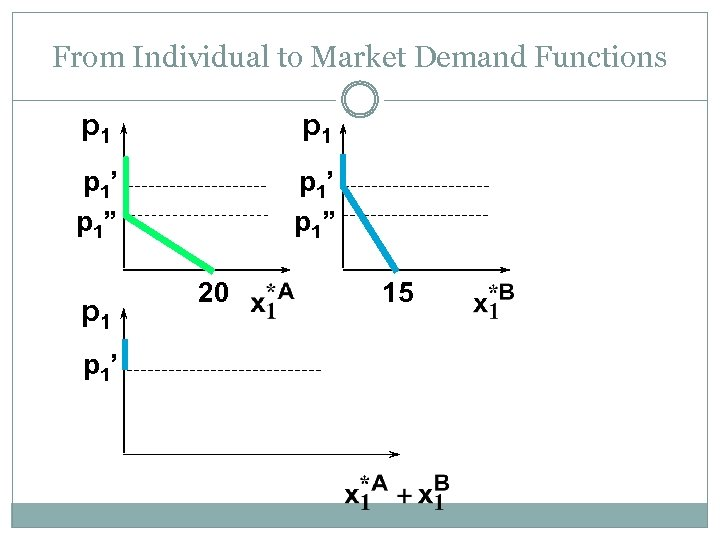From Individual to Market Demand Functions p 1 p 1’ p 1” p 1’ 20 15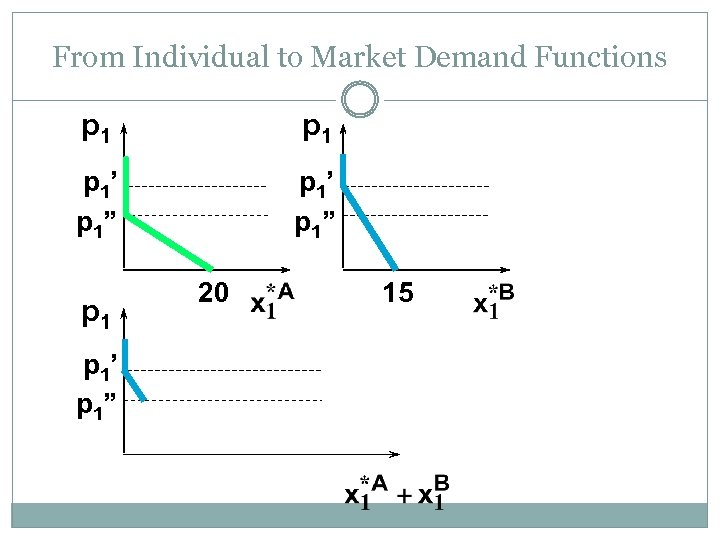From Individual to Market Demand Functions p 1 p 1’ p 1” 20 15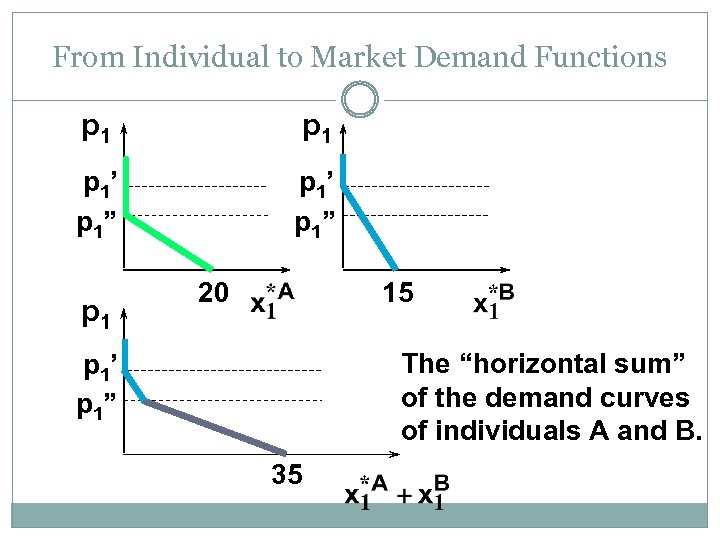From Individual to Market Demand Functions p 1 p 1’ p 1” p 1 20 15 The “horizontal sum” of the demand curves of individuals A and B. p 1’ p 1” 35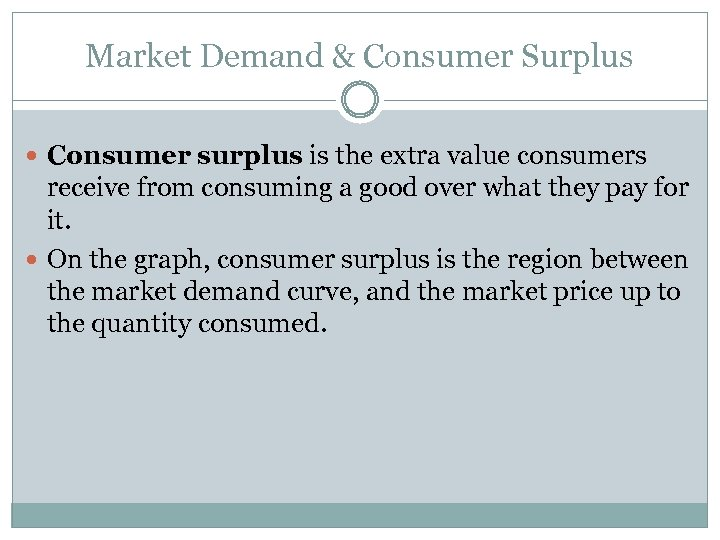Market Demand & Consumer Surplus Consumer surplus is the extra value consumers receive from consuming a good over what they pay for it. On the graph, consumer surplus is the region between the market demand curve, and the market price up to the quantity consumed.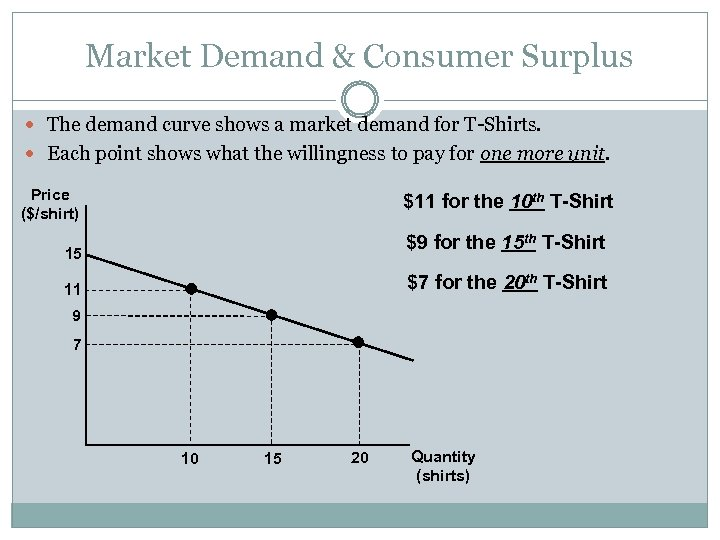Market Demand & Consumer Surplus The demand curve shows a market demand for T-Shirts. Each point shows what the willingness to pay for one more unit. Price (\$/shirt) \$11 for the 10 th T-Shirt \$9 for the 15 th T-Shirt 15 \$7 for the 20 th T-Shirt 11 9 7 10 15 20 Quantity (shirts)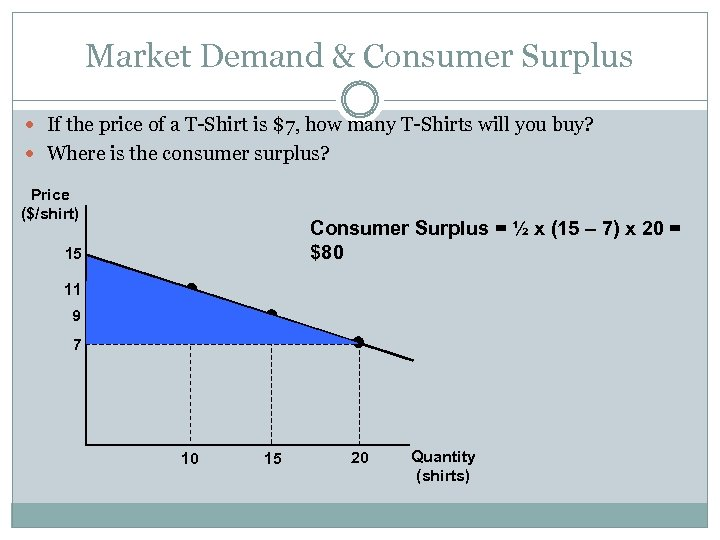Market Demand & Consumer Surplus If the price of a T-Shirt is \$7, how many T-Shirts will you buy? Where is the consumer surplus? Price (\$/shirt) Consumer Surplus = ½ x (15 – 7) x 20 = \$80 15 11 9 7 10 15 20 Quantity (shirts)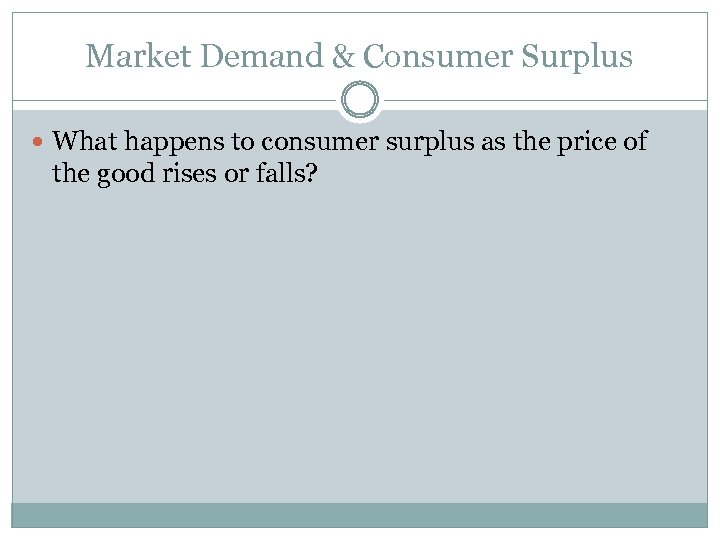Market Demand & Consumer Surplus What happens to consumer surplus as the price of the good rises or falls?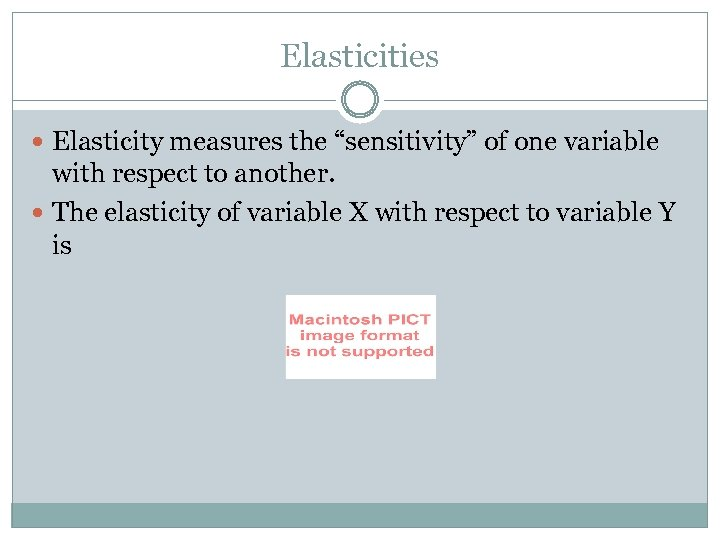Elasticities Elasticity measures the “sensitivity” of one variable with respect to another. The elasticity of variable X with respect to variable Y is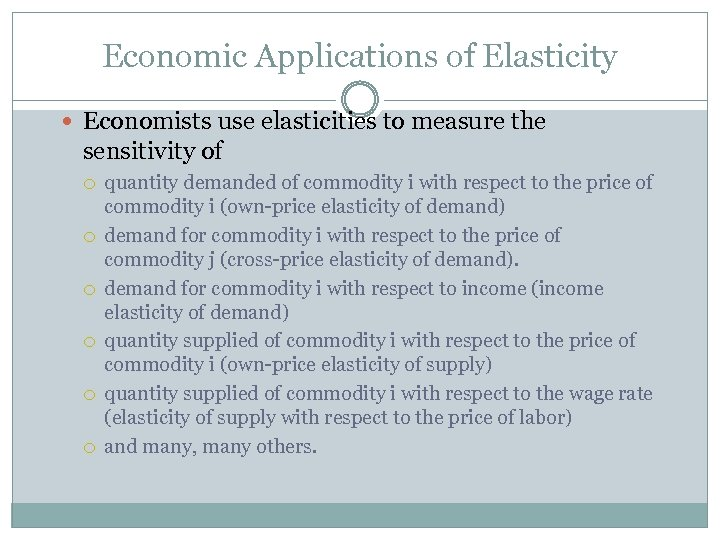Economic Applications of Elasticity Economists use elasticities to measure the sensitivity of quantity demanded of commodity i with respect to the price of commodity i (own-price elasticity of demand) demand for commodity i with respect to the price of commodity j (cross-price elasticity of demand). demand for commodity i with respect to income (income elasticity of demand) quantity supplied of commodity i with respect to the price of commodity i (own-price elasticity of supply) quantity supplied of commodity i with respect to the wage rate (elasticity of supply with respect to the price of labor) and many, many others.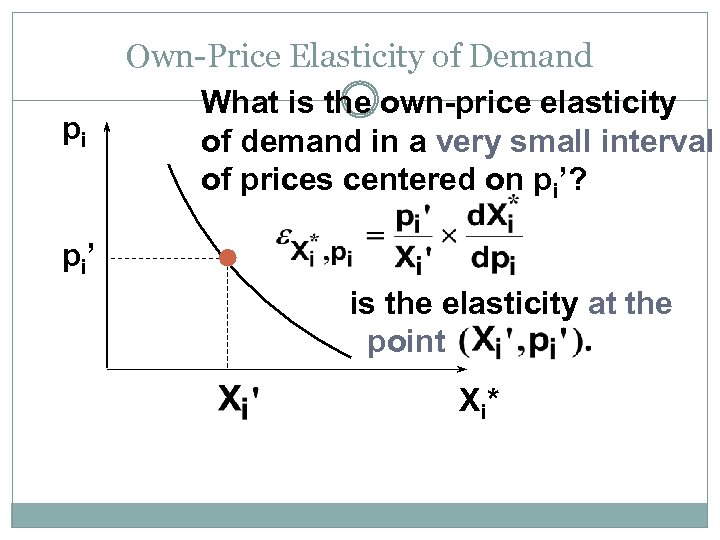pi Own-Price Elasticity of Demand What is the own-price elasticity of demand in a very small interval of prices centered on pi’? p i’ is the elasticity at the point X i*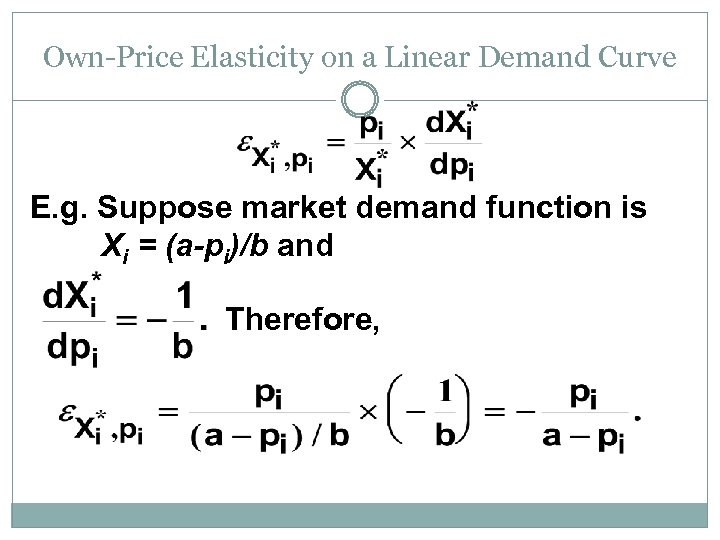Own-Price Elasticity on a Linear Demand Curve E. g. Suppose market demand function is Xi = (a-pi)/b and Therefore,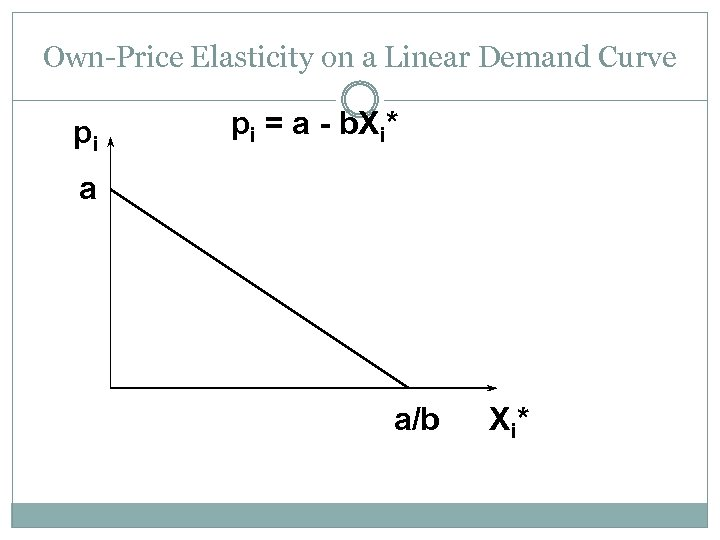Own-Price Elasticity on a Linear Demand Curve pi pi = a - b. Xi* a a/b X i*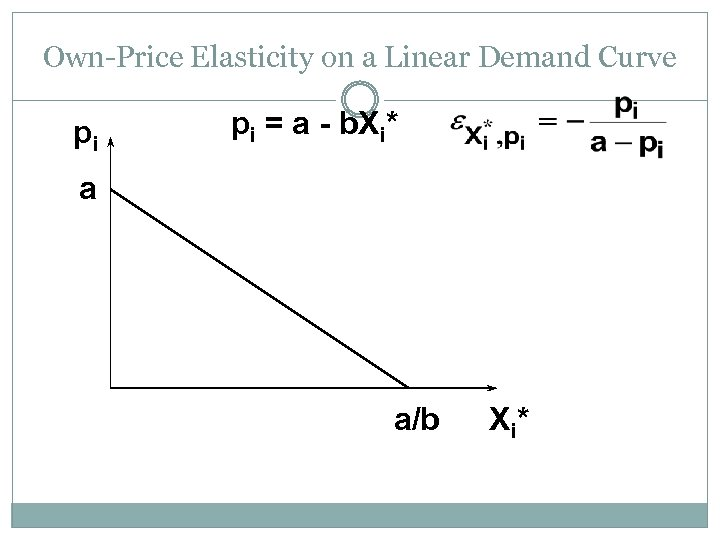Own-Price Elasticity on a Linear Demand Curve pi pi = a - b. Xi* a a/b X i*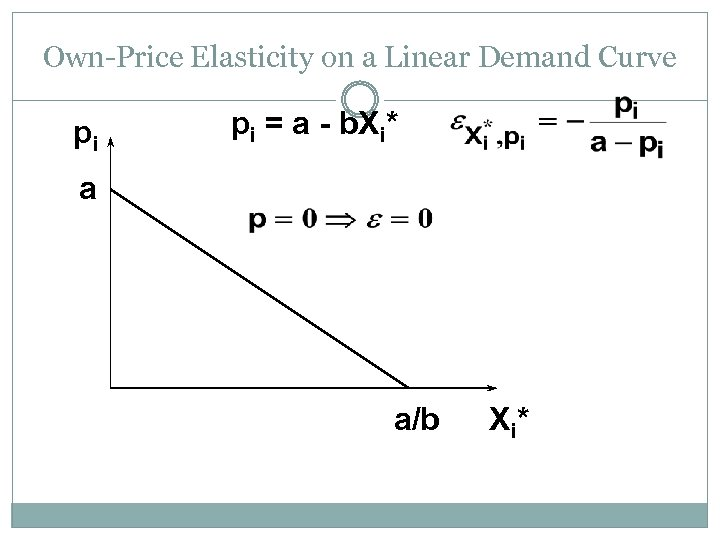Own-Price Elasticity on a Linear Demand Curve pi pi = a - b. Xi* a a/b X i*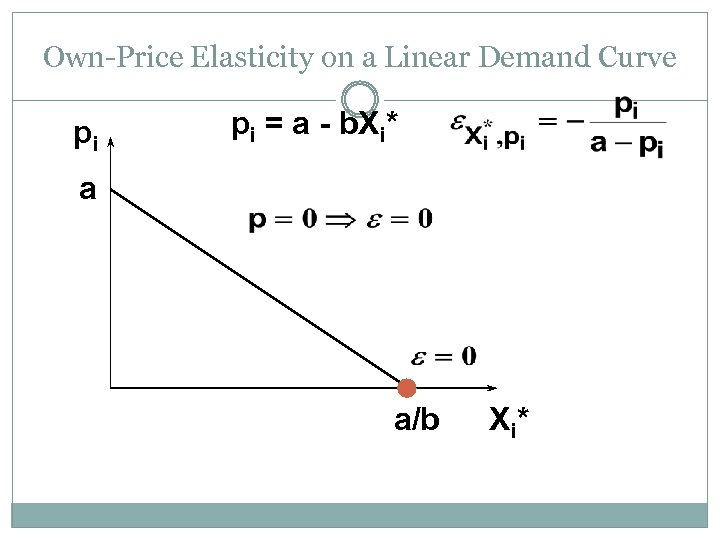Own-Price Elasticity on a Linear Demand Curve pi pi = a - b. Xi* a a/b X i*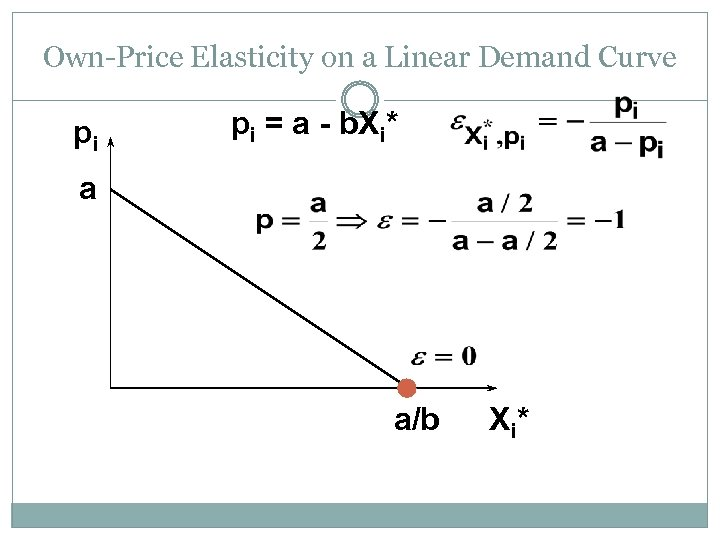Own-Price Elasticity on a Linear Demand Curve pi pi = a - b. Xi* a a/b X i*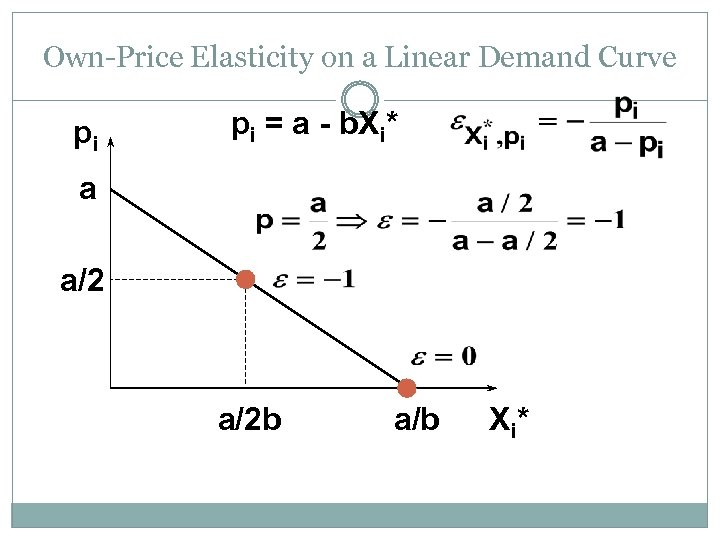Own-Price Elasticity on a Linear Demand Curve pi pi = a - b. Xi* a a/2 b a/b X i*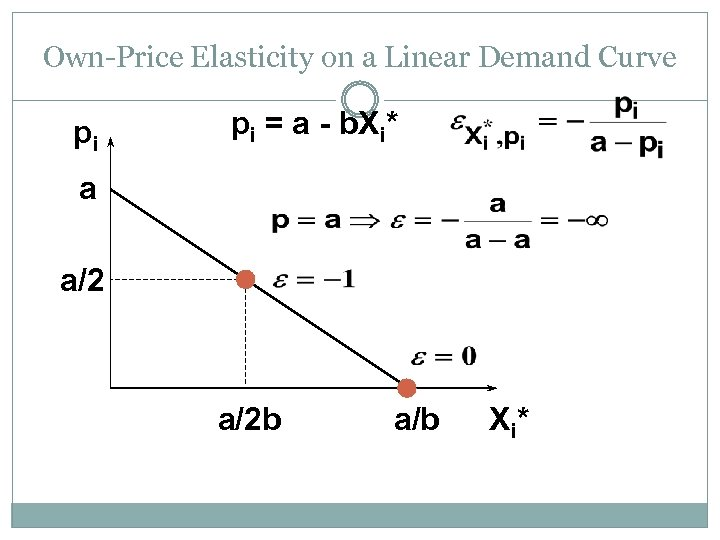Own-Price Elasticity on a Linear Demand Curve pi pi = a - b. Xi* a a/2 b a/b X i*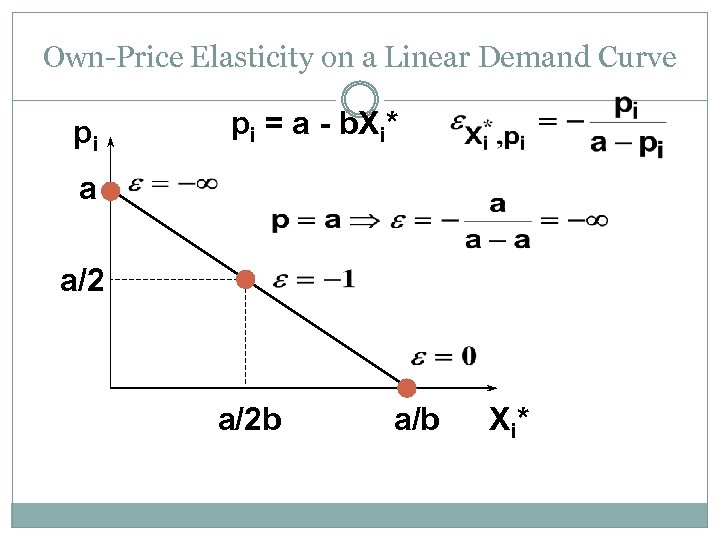Own-Price Elasticity on a Linear Demand Curve pi pi = a - b. Xi* a a/2 b a/b X i*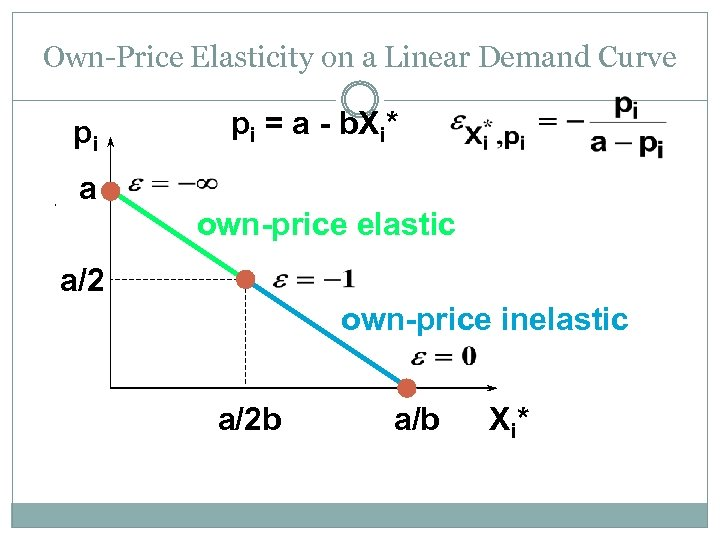Own-Price Elasticity on a Linear Demand Curve pi a pi = a - b. Xi* own-price elastic a/2 own-price inelastic a/2 b a/b X i*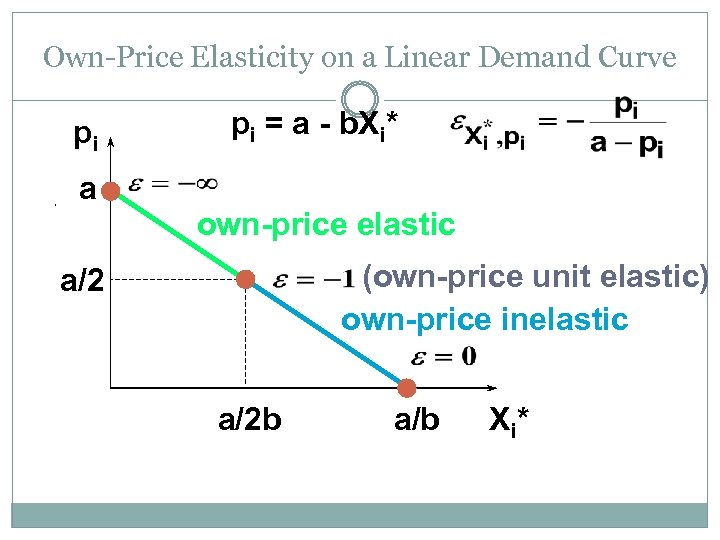Own-Price Elasticity on a Linear Demand Curve pi a pi = a - b. Xi* own-price elastic (own-price unit elastic) own-price inelastic a/2 b a/b X i*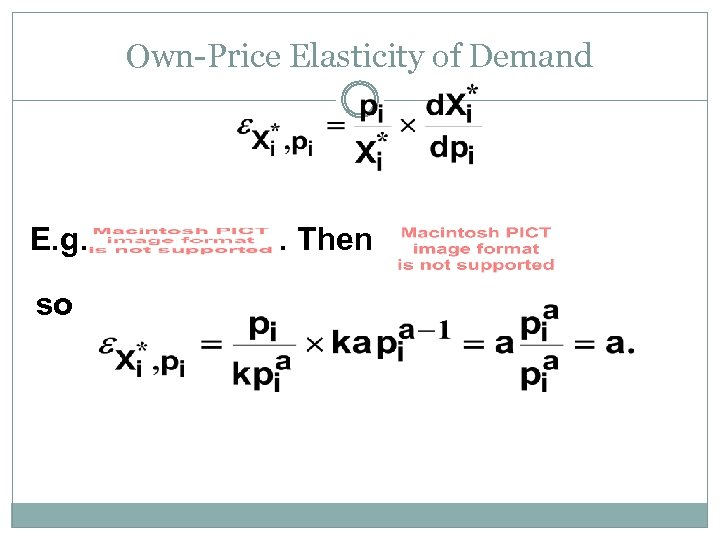Own-Price Elasticity of Demand E. g. so . Then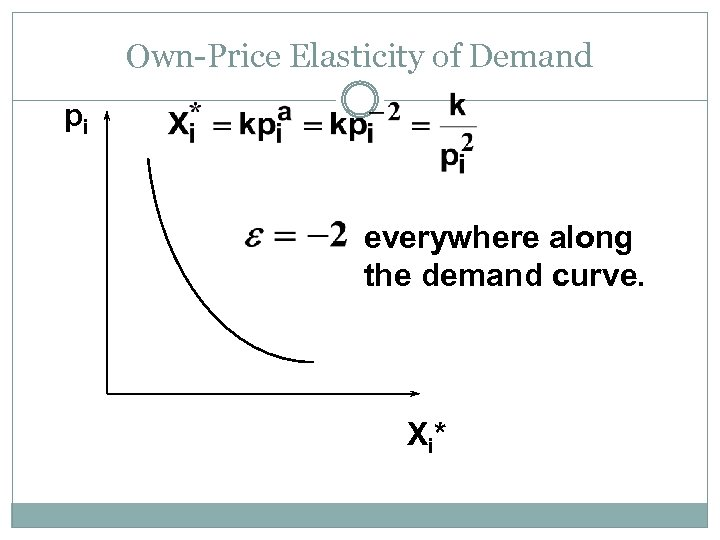Own-Price Elasticity of Demand pi everywhere along the demand curve. X i*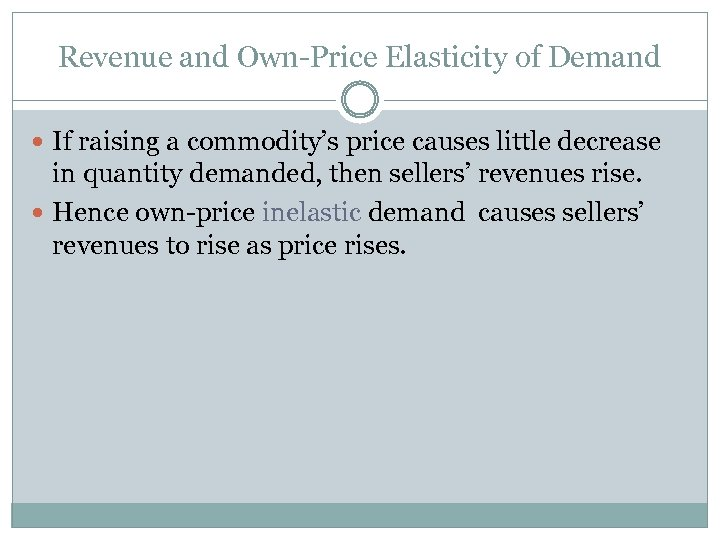Revenue and Own-Price Elasticity of Demand If raising a commodity’s price causes little decrease in quantity demanded, then sellers’ revenues rise. Hence own-price inelastic demand causes sellers’ revenues to rise as price rises.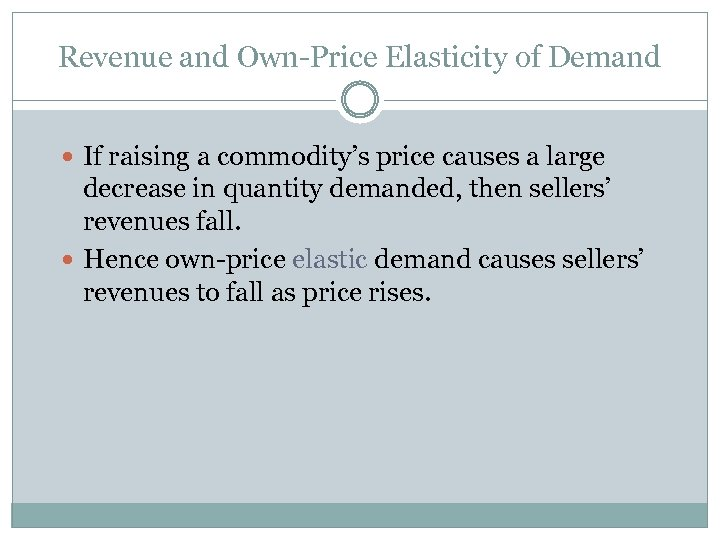Revenue and Own-Price Elasticity of Demand If raising a commodity’s price causes a large decrease in quantity demanded, then sellers’ revenues fall. Hence own-price elastic demand causes sellers’ revenues to fall as price rises.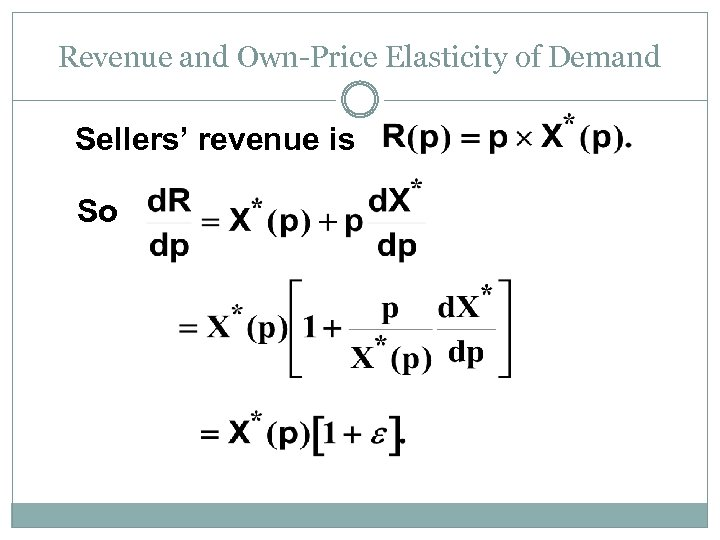Revenue and Own-Price Elasticity of Demand Sellers’ revenue is So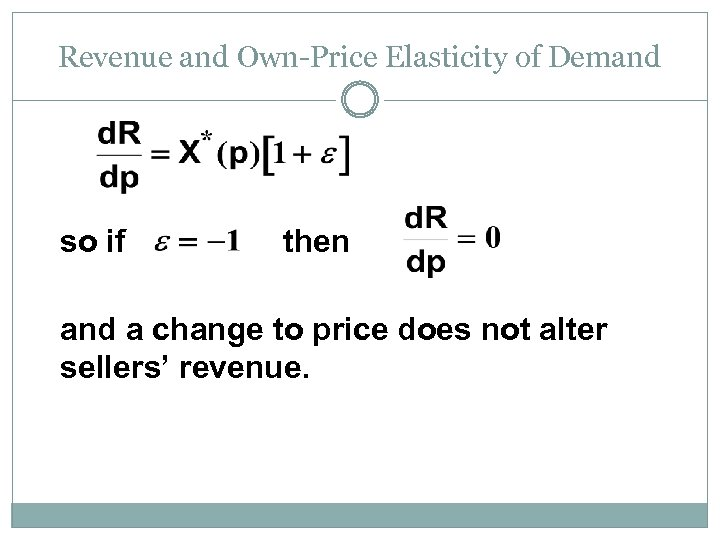Revenue and Own-Price Elasticity of Demand so if then and a change to price does not alter sellers’ revenue.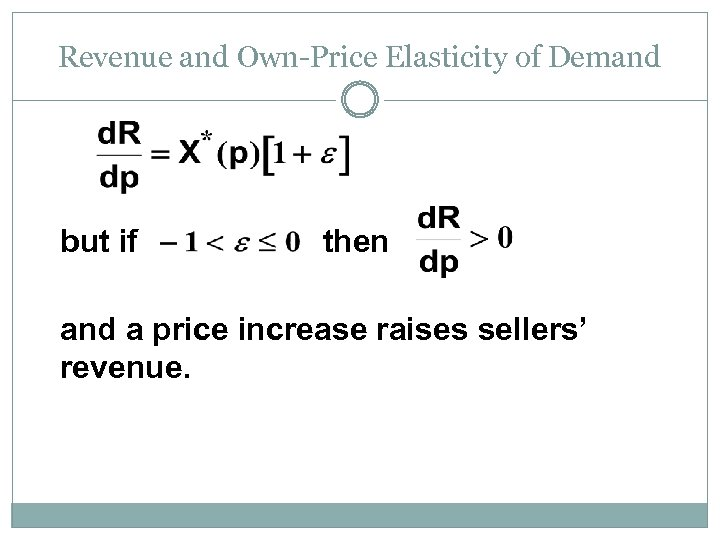Revenue and Own-Price Elasticity of Demand but if then and a price increase raises sellers’ revenue.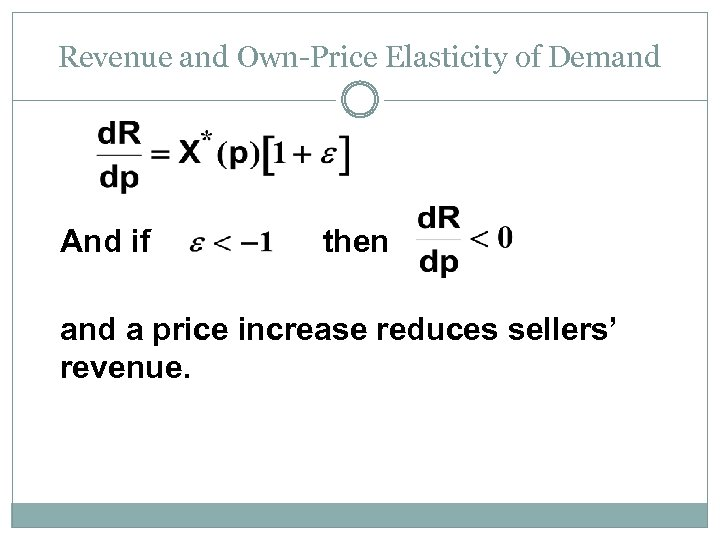Revenue and Own-Price Elasticity of Demand And if then and a price increase reduces sellers’ revenue.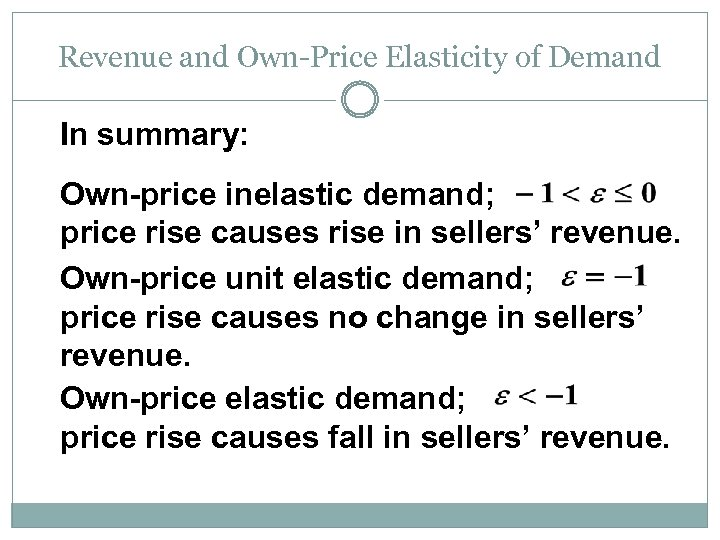Revenue and Own-Price Elasticity of Demand In summary: Own-price inelastic demand; price rise causes rise in sellers’ revenue. Own-price unit elastic demand; price rise causes no change in sellers’ revenue. Own-price elastic demand; price rise causes fall in sellers’ revenue.